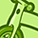# QlikView App Dev

Discussion Board for collaboration related to QlikView App Development.

cancel
Showing results for
Did you mean:Contributor III

## zero values in aggr() function

Hi all,

I have some product and sales data. I created 2 straight table in which I am showing only 4 products sales at a time. see the picture below.

when I click next button those 2 straight tables show next 8 products.

for that in the dimensions of the straight table I am using below expressions

Straight table 1 caluculated dimension:

=if(Aggr(rank(sum({<Year = {'\$(=vCurrentYear)'}>}Sales), 4), [Product Name])> ((8 * \$(vTemp)) -8)

and

Aggr(rank(sum({<Year = {'\$(=vCurrentYear)'}>}Sales), 4), [Product Name])< ((8 * \$(vTemp)) - 3), [Product Name])

Straight table 2 caluculated dimension:

=if(Aggr(rank(sum({<Year = {'\$(=vCurrentYear)'}>}Sales), 4), [Product Name])>= ((8 * \$(vTemp)) - 3)

and

Aggr(rank(sum({<Year = {'\$(=vCurrentYear)'}>}Sales), 4), [Product Name])< ((8 * \$(vTemp)) + 1), [Product Name])

It worked good so far, but there is a small problem.

If a product don't have any sales in a year then that product is not showing up in the straight table at all. I want to show all the products in the straight table even if it's sales are zero.

Thank you.

1 Solution

Accepted SolutionsMVP

Try something like

=if(

Aggr(rank(sum({<Year = {'\$(=vCurrentYear)'}>}Sales) +Sum({1} 0) , 4), [Product Name])> ((8 * \$(vTemp)) -8)

and

Aggr(rank(sum({<Year = {'\$(=vCurrentYear)'}>}Sales)+Sum({1} 0), 4), [Product Name])< ((8 * \$(vTemp)) - 3)

, [Product Name])

6 RepliesMVP

Can you make sure that 'Suppress When Zero' is unchecked on the presentation tab?MVP

Try something like

=if(

Aggr(rank(sum({<Year = {'\$(=vCurrentYear)'}>}Sales) +Sum({1} 0) , 4), [Product Name])> ((8 * \$(vTemp)) -8)

and

Aggr(rank(sum({<Year = {'\$(=vCurrentYear)'}>}Sales)+Sum({1} 0), 4), [Product Name])< ((8 * \$(vTemp)) - 3)

, [Product Name])Contributor III
Author

Yes sunny, I unchecked 'Supress Zero values' option. still it is not working.

Thank you.Contributor III
Author

Thank you swuehl, Your answer is working fine.Creator III

HI Swuehl,

I am facing similar issue , could you help me please.

i am writing below expression

Num(Avg(Aggr(Avg([SVT GDC TAT NumValue]), [SVT GDC TAT ID])),'#,##0' ) when i do so values of zero is getting suppressed but where as i need Zero values as well for [SVT GDC TAT ID].Creator III

HI Swuehl,

I am facing similar issue , could you help me please.

i am writing below expression

Num(Avg(Aggr(Avg([SVT GDC TAT NumValue]), [SVT GDC TAT ID])),'#,##0' )

when i do so values of zero is getting suppressed but where as i need Zero values as well for [SVT GDC TAT ID]